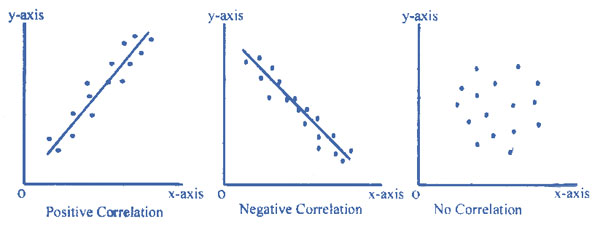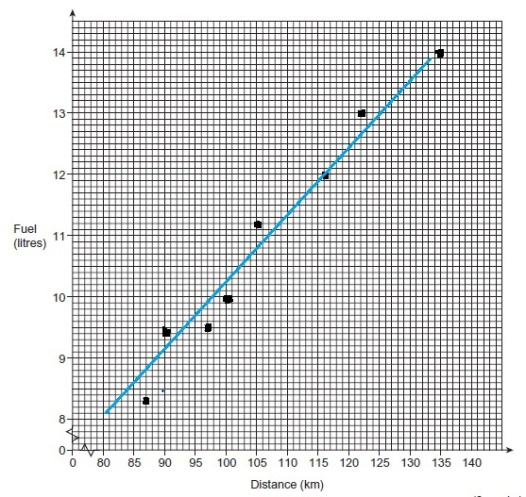# GSCE Maths: Scatterplot GraphsA scatterplot graph has a positive correlation if the results are approximately in a straight line with a positive slope (i.e. as one rises, the other rises too). It has a negative correlation if the results are approximately in a straight line with a negative slope (i.e. as one rises the other falls). If the points on a scatterplot are not aligned with each other, no correlation exists.

If a correlation exists then a ‘line of best fit’ can be drawn, this is a line that best aligns the points – i.e. any points not on the line should be evenly distributed on either side of the line.

Sample question

Each day a taxi driver records the distance he travels and the amount of fuel his car uses.

 Distance (km) 87 122 97 90 105 100 135 116 Fuel (litres) 8.3 13 9.5 9.4 11.2 9.9 14 12

i) Complete the scatter graph and draw a line of best fit.

ii) Use your line of best fit to predict the fuel used to travel 110 km.

Solutioni) The scatterplot shows a positive correlation between distance travelled and fuel used; so a line of best fit can be drawn. (i.e. the solid blue solid line)

ii) From the scatterplot, it can be seen that travelling 110km corresponds to a fuel use of approximately 11.3 litres.

### Work Towards That 'A' Grade

Achieve the results you want with-written revision resources when you register with iRevise.
Its Free!